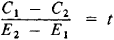# Payoff on Capital Investment

The following article is from The Great Soviet Encyclopedia (1979). It might be outdated or ideologically biased.

## Payoff on Capital Investment

one of the indexes of the effectiveness of capital investments. The payoff is the ratio of capital investment to the economic effect obtained through the investment.

In the USSR the goal of capital investment in building new industrial enterprises and rebuilding and expanding existing ones is to increase the volume of production and raise the productivity of social labor, which determines the pace of the building of communism. “The party attaches prime importance to raising the effectiveness of capital investments, selecting the most profitable and economical areas for capital work, ensuring maximum growth in output per ruble of capital investment, and reducing payoff periods for these investments” (Programma KPSS, 1973, p. 86). On the scale of the entire national economy the effect of capital investments is measured by increases in net output, or in national income. The ratio of capital investments to the average annual growth in national income caused by these investments is equal to the payoff period, expressed in years. Thus the general formula for payoff in the national economy is

C/ ΔI = t

where C is capital investments, ΔI is the growth in national income caused by the investments, and t is the payoff period. If the portion of national income resulting from the particular capital investment can be determined, payoff on capital investment can be determined quite precisely for the national economy as a whole.

Calculating payoff on capital investment by sectors of the national economy produces a less exact result because current prices deviate from cost. Therefore, when the national income is computed on a sector-by-sector basis, the calculated figure also deviates from the true magnitude. In practice, contribution to national income is not computed at all within industrial sectors and individual enterprises. Hence in these cases the payoff on capital investments is provisionally determined by comparing capital investment with growth in annual profits or with yearly savings in prime cost of output resulting from capital investment:

C/ ΔP = t

where Δp is growth in profits equal to savings in prime cost. Because profit growth is usually less than growth in net output, the payoff period for profit growth is also less.

Thus, payoff on capital investment is computed on the basis of the magnitude of the absolute effect in the form of growth in income or growth in net output and in the form of profit growth and reduction of current expenditures.

When choosing the optimal variant among investment choices, payoff on capital investment may be used as an index of comparative effectiveness. Variant solutions to a given economic problem, such as how best to develop a particular sector or enterprise, usually require different capital investments and current expenditures. Moreover, acceptable variants that require substantial investment will have lower prime cost. (If a variation with greater capital investments also has higher prime cost, it is plainly disadvantageous.) A paired comparison of variants for capital investment and current expenditure is made to select the optimal variant. For an arbitrarily selected pair the better variant, that is, the one that produces a shorter payoff period, is determined. This better variant is then compared with any other by the chain method, according to which the better of these two is determined and so on until the best variant of all those considered has been found.

As a result of such calculations, the comparative effectiveness of variants can be determined by examining the ratio of the difference of capital investments and the difference of current expenditures according to the expressionwhere t is the payoff period in years, C1 and C2 are capital investments for a pair of variants being compared, and E1 and E2 are current expenditures. If the payoff period obtained is lower than the established standard to, then variant C1 is recognized as being more effective than C2.

Where there are a large number of variants the calculation is not ordinarily done by using payoff on capital investment. Instead the minimum of calculated expenditures is used, and the quantity 1/to, the inverse of the payoff period, is employed as a standard. If the investment is to replace machinery, the indexes for the existing machinery are considered as one of the variants being compared.

### REFERENCES

Khachaturov, T. S. Ekonomicheskaia effektivnost’kapital’nykh vlozhenii. Moscow, 1964.
Bogachev, V. N. “Srok okupaemosti.” Teoriia sravneniia planovykh variantov. Moscow, 1966.
Novozhilov, V. V. Problemy izmereniia zatral i rezul’tatov pri optimal’nom planirovanii. Moscow, 1967.
Krasnovskii, V. P. Problemy ekonomiki kapital’nykh vlozhenii. Moscow, 1967.
Voprosy izmereniia effektivnosti kapital’nykh vlozhenii. Moscow, 1968. Tipovaia metodika opredeleniia effektivnosti kapital’nykh vlozhenii. Moscow, 1969.
Masse, P. Kriterii i melody optimal’nogo opredeleniia kapitalovlozhenii. Moscow, 1971. (Translated from French.)

T. S. KHACHATUROV